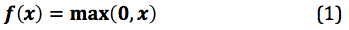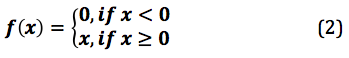# ReLu

## What is ReLu?

ReLu is a non-linear activation function that is used in multi-layer neural networks or deep neural networks. This function can be represented as:where x = an input value

According to equation 1, the output of ReLu is the maximum value between zero and the input value. An output is equal to zero when the input value is negative and the input value when the input is positive. Thus, we can rewrite equation 1 as follows:where x = an input value

## Examples of ReLu

Given different inputs, the function generates different outputs. For example, when x is equal to -5, the output of f(-5) is 0. By contrast, the output of f(0) is 0 because the input is greater or equal to 0. Further, the result of f(5) is 5 because the input is greater than zero.

## The Purpose of ReLu

Traditionally, some prevalent non-linear activation functions, like sigmoid functions (or logistic) and hyperbolic tangent, are used in neural networks to get activation values corresponding to each neuron. Recently, the ReLu function has been used instead to calculate the activation values in traditional neural network or deep neural network paradigms. The reasons of replacing sigmoid and hyperbolic tangent with ReLu consist of:

1. Computation saving - the ReLu function is able to accelerate the training speed of deep neural networks compared to traditional activation functions since the derivative of ReLu is 1 for a positive input. Due to a constant, deep neural networks do not need to take additional time for computing error terms during training phase.